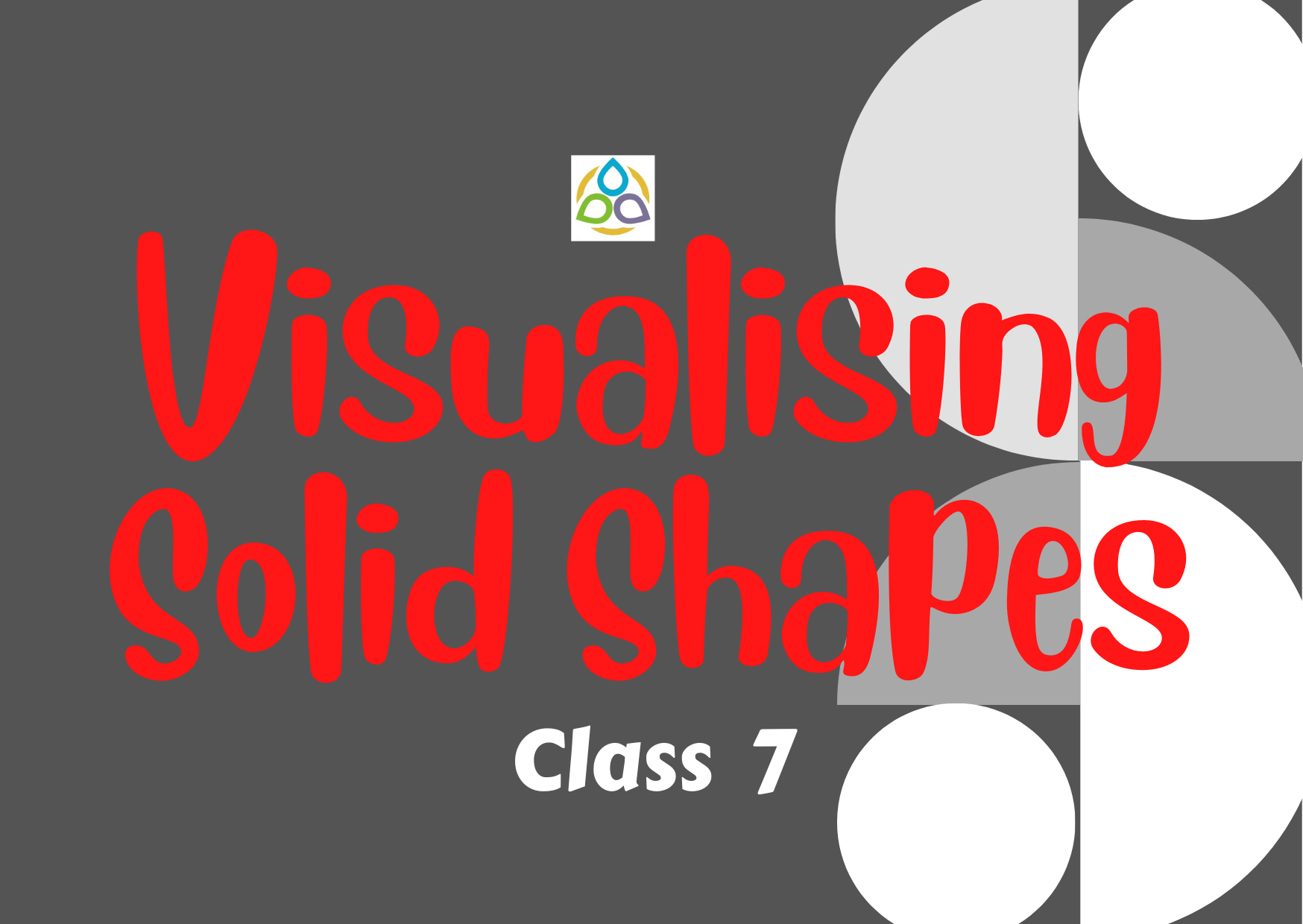# Visualising Solid Shapes – 7

45. Total number of edges a cylinder has

• (A) 0
• (B) 1
• (C) 2
• (D) 3

44. A solid that has two opposite identical faces and other faces as parallelograms is a

• (A) Prism
• (B) Pyramid
• (C) Cone
• (D) Sphere

43. The number of triangular faces in a tetrahedron is

• (A) 5
• (B) 4
• (C) 2
• (D) 3

42. The solid with one circular face, one curved surface and one vertex is known as

• (A) Cone
• (B) Sphere
• (C) Cylinder
• (D) Prism

41. When we cut a corner of a cube as shown in the following figure, we get the cutout piece as

• (A) Square pyramid
• (B) Trapezium prism
• (C) Triangular pyramid
• (D) A triangle

40. When a torch is pointed towards one of the vertical edges of a cube, we get a shadow of the cube in the shape of

• (A) square
• (B) rectangle but not a square
• (C) circle
• (D) triangle

39. Each face of a cuboid is a

• (A) Triangle
• (B) Square
• (C) Rectangle
• (D) Rhombus

38. Isometric sheet divides the paper into small triangles made up of dots or lines. The shape of these triangles is

• (A) Isosceles
• (B) Equilateral
• (C) Scalene
• (D) None of these

37. How many edges are there in a pentagonal prism?

• (A) 16
• (B) 15
• (C) 18
• (D) 12

36. Which of the following three dimensional figures has the top, side and front as triangles?

• (A) (i)
• (B) (ii)
• (C) (iii)
• (D) (iv)

35. Which of the following is the top view of the given shape?

• (A) (i)
• (B) (ii)
• (C) (iii)
• (D) (iv)

34. Which amongst the following is not a polyhedron?

• (A) (i)
• (B) (ii)
• (C) (iii)
• (D) (iv)

33. The net shown below can be folded into the shape of a cube. The face marked with the letter L is opposite to the face marked with which letter?

• (A) M
• (B) N
• (C) Q
• (D) O

32. What is the value of F + VE in the following figure?

• (A) 1
• (B) 2
• (C) 4
• (D) 3

31. What is the value of F + VE in the following figure?

• (A) 1
• (B) 2
• (C) 4
• (D) 3